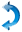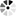#### ViaTrains Between Stations

 FromTo

#### Arrival/Departure at Station

 ALL LIVE

#### Recent Search History

 No Recent Search History.
 Welcome to etrain.info :-) 09-Jul-2020 06:53:59 IST

#### Arrival / Departure at Station - NEW DELHI (NDLS)

 TrainNumber Train Name SourceStation Dest.Station Train Timings Running Days Available Classes Arrival Depart. Halt Su Mo Tu We Th Fr Sa 1A FC 2A 3A 3E CC SL 2S
 02801 PURUSHOTTAM EXP BBS NDLS 04:20 -- -- Y Y Y Y Y Y Y X X 2A 3A X X SL 2S 02391 SHRAMJEEVI EXP RGD NDLS 04:45 -- -- Y Y Y Y Y Y Y 1A X 2A 3A X X SL 2S 02426 JAT NDLS SPL JAT NDLS 05:00 -- -- Y Y Y Y Y Y Y 1A X 2A 3A X X X X 02565 BIHAR S KRANTI DBG NDLS 05:30 -- -- Y Y Y Y Y Y Y 1A X 2A 3A X X SL 2S 02691 SBC NDLS AC SPL SBC NDLS 05:55 -- -- Y Y Y Y Y Y Y 1A X 2A 3A X X X X 02303 POORVA EXPRESS HWH NDLS 06:05 -- -- Y X Y Y X X Y 1A X 2A 3A X X SL 2S 02381 POORVA EXPRESS HWH NDLS 06:05 -- -- X Y X X Y Y X 1A X 2A 3A X X SL 2S 02555 GORAKDAM EXPRES GKP HSR 05:50 06:05 00:15H Y Y Y Y Y Y Y 1A X 2A 3A X X SL 2S 02451 SHRAM SHKTI EXP CNB NDLS 06:30 -- -- Y Y Y Y Y Y Y 1A X 2A 3A X X SL 2S 02805 VSKP NDLS AP EXP VSKP NDLS 06:35 -- -- Y Y Y Y Y Y Y 1A X 2A 3A X X SL 2S 02553 VAISHALI EXP SHC NDLS 06:40 -- -- Y Y Y Y Y Y Y 1A X 2A 3A X X SL 2S 02229 LUCKNOW MAIL LJN NDLS 06:50 -- -- Y Y Y Y Y Y Y 1A X 2A 3A X X SL 2S 02417 PRAYAG RAJ EXP PRYJ NDLS 07:00 -- -- Y Y Y Y Y Y Y 1A X 2A 3A X X SL 2S 02309 RJPB NDLS AC SPL RJPB NDLS 07:40 -- -- Y Y Y Y Y Y Y 1A X 2A 3A X X X X 02393 S KRANTI SUP EX RJPB NDLS 07:50 -- -- Y Y Y Y Y Y Y 1A X 2A 3A X X SL 2S 02957 NDLS RAJ SPL ADI NDLS 08:00 -- -- Y Y Y Y Y Y Y 1A X 2A 3A X X X X 02559 SHIV GANGA EXP MUV NDLS 08:10 -- -- Y Y Y Y Y Y Y 1A X 2A 3A X X SL 2S 02217 MAHAMANA EXP BSB NDLS 08:20 -- -- Y X X Y X Y X 1A X 2A X X X SL 2S 02723 TELANGANA EXP HYB NDLS 09:05 -- -- Y Y Y Y Y Y Y 1A X 2A 3A X X SL 2S 02951 MMCT NDLS AC SPL MMCT NDLS 09:05 -- -- Y Y Y Y Y Y Y 1A X 2A 3A X X X X 02301 HWH NDLS AC SPL HWH NDLS 10:00 -- -- Y Y Y Y Y Y Y 1A X 2A 3A X X X X 02423 DBRG NDLS AC SPL DBRG NDLS 10:10 -- -- Y Y Y Y Y Y Y 1A X 2A 3A X X X X 02433 MAS NDLS EXP MAS NDLS 10:30 -- -- Y X X X Y X X 1A X 2A 3A X X X X 02437 SC NDLS AC SPL SC NDLS 10:40 -- -- X X X X Y X X 1A X 2A 3A X X X X 02823 BBS NDLS AC SPL BBS NDLS 10:45 -- -- Y Y Y Y Y Y Y 1A X 2A 3A X X X X 02453 RNC NDLS RAJ SPL RNC NDLS 10:55 -- -- X Y X X X Y X 1A X 2A 3A X X X X 02441 BSP NDLS SPL BSP NDLS 10:55 -- -- X X Y X X Y X 1A X 2A 3A X X X X 02925 PASCHIM EXPRESS BDTS KLK 10:40 11:05 00:25H Y Y Y Y Y Y Y X X 2A 3A X X SL 2S 02925 PASCHIM EXPRESS BDTS ASR 10:40 11:05 00:25H Y Y Y Y Y Y Y 1A X 2A 3A X X SL 2S 02056 NDLS JANSHATABDI DDN NDLS 11:15 -- -- Y Y Y Y Y Y Y X X X X X CC X 2S 02432 NDLS TVC SPL NDLS TVC -- 11:25 -- Y X Y Y X X X 1A X 2A 3A X X X X 02414 NDLS MAO AC SPL NDLS MAO -- 11:25 -- X X X X X Y Y 1A X 2A 3A X X X X 02501 AGTL NDLS AC SPL AGTL NDLS 11:40 -- -- X X X Y X X X 1A X 2A 3A X X X X 02058 NDLS JANSHTBDI UHL NDLS 12:00 -- -- Y Y Y Y Y Y Y X X X X X CC X 2S 02420 GOMTI EXPRESS NDLS LKO -- 12:25 -- Y Y Y Y Y Y Y 1A X 2A X X CC X 2S 02715 SACHKHAND EXP NED ASR 12:10 12:30 00:20H Y Y Y Y Y Y Y X X 2A 3A X X SL 2S 02413 MAO NDLS RAJ SPL MAO NDLS 12:40 -- -- X Y Y X X X X 1A X 2A 3A X X X X 02392 SHRAMJEVI N EXP NDLS RGD -- 13:10 -- Y Y Y Y Y Y Y 1A X 2A 3A X X SL 2S 02716 ASR NED EXPRESS ASR NED 13:00 13:20 00:20H Y Y Y Y Y Y Y X X 2A 3A X X SL 2S 02566 BIHAR S KRANTI NDLS DBG -- 14:15 -- Y Y Y Y Y Y Y 1A X 2A 3A X X SL 2S 02057 UHL JANSHATABDI NDLS UHL -- 14:35 -- Y Y Y Y Y Y Y X X X X X CC X 2S 02419 GOMTI EXP LKO NDLS 15:05 -- -- Y Y Y Y Y Y Y 1A X 2A X X CC X 2S 02055 DDN JANSHTBDI NDLS DDN -- 15:20 -- Y Y Y Y Y Y Y X X X X X CC X 2S 02454 NDLS RNC SPL NDLS RNC -- 15:30 -- X X X Y X X Y 1A X 2A 3A X X X X 02442 NDLS BSP SPECIAL NDLS BSP -- 16:00 -- X X Y X X X Y 1A X 2A 3A X X X X 02438 NDLS SC SPL NDLS SC -- 16:00 -- Y X X X X X X 1A X 2A 3A X X X X 02434 NDLS MAS SPL NDLS MAS -- 16:00 -- X Y X X Y X X 1A X 2A 3A X X X X 02424 NDLS DBRG SPL NDLS DBRG -- 16:10 -- Y Y Y Y Y Y Y 1A X 2A 3A X X X X 02926 PASCHIM EXPRESS ASR BDTS 16:25 16:45 00:20H Y Y Y Y Y Y Y 1A X 2A 3A X X SL 2S 20926 PASCHIM EXP SPL KLK BDTS 16:25 16:45 00:20H Y Y Y Y Y Y Y X X 2A 3A X X SL 2S 02302 NDLS HWH AC SPL NDLS HWH -- 16:55 -- Y Y Y Y Y Y Y 1A X 2A 3A X X X X 02431 TVC NDLS EXP TVC NDLS 16:55 -- -- Y X X X Y X Y 1A X 2A 3A X X X X 02952 NDLS MMCT AC SPL NDLS MMCT -- 17:00 -- Y Y Y Y Y Y Y 1A X 2A 3A X X X X 02824 NDLS BBS AC EXP NDLS BBS -- 17:05 -- Y Y Y Y Y Y Y 1A X 2A 3A X X X X 02310 NDLS RJPB AC SPL NDLS RJPB -- 17:15 -- Y Y Y Y Y Y Y 1A X 2A 3A X X X X 02394 SAMPOORN K EXP NDLS RJPB -- 17:25 -- Y Y Y Y Y Y Y 1A X 2A 3A X X SL 2S 02724 TELANGANA EXP NDLS HYB -- 17:25 -- Y Y Y Y Y Y Y 1A X 2A 3A X X SL 2S 02382 POORVA EXP SPL NDLS HWH -- 17:35 -- X Y Y X X Y X 1A X 2A 3A X X SL 2S 02304 POORVA EXPRESS NDLS HWH -- 17:35 -- Y X X Y Y X Y 1A X 2A 3A X X SL 2S 02218 MAHAMANA EXP NDLS BSB -- 18:35 -- X Y X Y X Y X 1A X 2A X X X SL 2S 02560 SHIV GANGA EXP NDLS MUV -- 18:55 -- Y Y Y Y Y Y Y 1A X 2A 3A X X SL 2S 02554 VAISHALI EXP NDLS SHC -- 19:50 -- Y Y Y Y Y Y Y 1A X 2A 3A X X SL 2S 02502 NDLS AGTL AC SPL NDLS AGTL -- 19:50 -- X X X Y X X X 1A X 2A 3A X X X X 02806 NDLS VSKP AP EXP NDLS VSKP -- 20:15 -- Y Y Y Y Y Y Y 1A X 2A 3A X X SL 2S 02958 RAJDHANI SPL NDLS ADI -- 20:25 -- Y Y Y Y Y Y Y 1A X 2A 3A X X X X 02556 GORAKDAM EXPRES HSR GKP 20:10 20:25 00:15H Y Y Y Y Y Y Y 1A X 2A 3A X X SL 2S 02425 NDLS JAT SPL NDLS JAT -- 21:10 -- Y Y Y Y Y Y Y 1A X 2A 3A X X X X 02692 NDLS SBC AC SPL NDLS SBC -- 21:15 -- Y Y Y Y Y Y Y 1A X 2A 3A X X X X 02418 PRAYAG RAJ EXP NDLS PRYJ -- 21:20 -- Y Y Y Y Y Y Y 1A X 2A 3A X X SL 2S 02230 LUCKNOW MAIL NDLS LJN -- 22:05 -- Y Y Y Y Y Y Y 1A X 2A 3A X X SL 2S 02802 PURSHOTTAM SPL NDLS BBS -- 22:25 -- Y Y Y Y Y Y Y X X 2A 3A X X SL 2S 02452 SHRAM SHKTI EXP NDLS CNB -- 23:55 -- Y Y Y Y Y Y Y 1A X 2A 3A X X SL 2S
 No. of Trains (Listed: 72 / Found: 72) No Filters AppliedLoading, Please Wait... QuickMenu#### Important Information

• Flexi Fare system applicable for Rajdhani, Duronto and Shatabdi trains. Know More
• Revised refund rules and changes in ticket cancellation charges w.e.f. 12-Nov-2015.
• Advance Reservation Period (ARP) has been increased to 120 Days w.e.f. 01 April 2015.

#### For Sub-Urban trains Information, Type and Select your Sub-Urban Station name and perform search.

Trains Information Available of Mumbai, Chennai, Hyderabad, Kolkata and Delhi Sub-Urban Trains.

#### Disclaimer

etrain.info is a private site and has no connection or affiliation with Indian Railways, Government of India or Government Railway sites in any way. All contents available in this website is just for Information Purpose and is intended to Personal Non-Commercial Use only.
Information available here like Arrival Departure of Trains at Stations, Train Route, Availability in Trains, PNR Status, Running Status, Running History, Rake Information or Fare Chart is collected from various sources and are subjected to change without any prior information. You are requested to re-verify it from other official sources before proceeding.